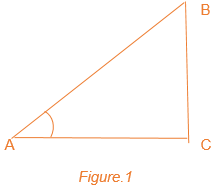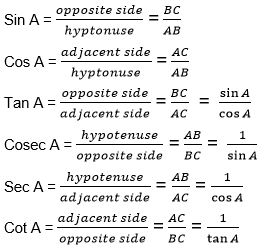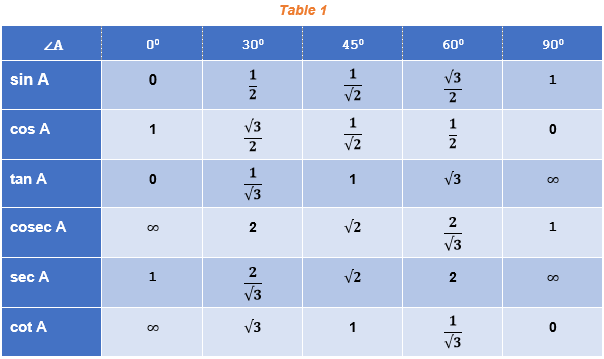Win up to 100% scholarship on Aakash BYJU'S JEE/NEET courses with ABNAT Win up to 100% scholarship on Aakash BYJU'S JEE/NEET courses with ABNAT

# General Solution of Trigonometric Equations

Trigonometry is derived from the Greek words trigonon (triangle) and metron (measure). It is a branch of Mathematics that deals with the relationships between the lengths and angles of the sides of triangles. A trigonometric equation is an equation involving one or more trigonometric ratios of unknown angles. It is expressed as ratios of sine(sin), cosine(cos), tangent(tan), cotangent(cot), secant(sec), and cosecant(cosec) angles. For example, cos2 x + 5 sin x = 0 is a trigonometric equation. All possible values which satisfy the given trigonometric equation are called solutions of the given trigonometric equation.

## Trigonometric Equations Formulas

Consider a right-angled triangle, as shown in Figure 1. From the figure, ∠A made is an acute angle.From the figure, the following trigonometric ratios can be deducted.Each of these trigonometric ratios can be evaluated at different angles. Some standard angles are given in Table 1 for each of the ratios. From these standard angles, we can extend the evaluation of trigonometric ratios for any angles.Remark: From Table 1 above, you can observe that as ∠ A increases from 0° to 90°, sin A increases from 0 to 1, and cos A decreases from 1 to 0.

Trigonometric ratios of complementary angles

From Figure 1, it is clear that ∠A + ∠B = 90° or ∠B = 90° – ∠A. So, consider the value for sine of ∠B, i.e., sin B = sin ( 90° – ∠A ) = AC/AB. But AC/AB turns out to be equal to cos A.

In short, we can have sin (90° – ∠A) = cos A. Similarly, we can define other trigonometric ratios for complementary angles as follows:

i. sin (90° – ∠A) = cos A

ii. cos (90° – ∠A) = sin A

iii. tan (90° – ∠A) = cot A

iv. cot (90° – ∠A) = tan A

v. sec (90° – ∠A) = cosec A

vi. cosec (90° – ∠A) = sec A.

Identities in Trigonometry

In Figure 1, it is clear that the right triangle ABC holds Pythagoras property as AB2 = AC2 + BC2

Dividing both sides by AB2, we get, 1 = cos2A + sin2A.

This is a very fundamental identity in trigonometry. Similarly, we can deduce other identities. They are listed below.

sin2A + cos2A = 1

sec2A – tan2A = 1

cosec2A – cot2A = 1

Trigonometric Formulas

There are some important formulas we must keep in mind to solve various trigonometric problems and situations. A few are given below:

cos (2nπ + x) = cos x

sin (2nπ + x) = sin x

cos (-x) = cos x

sin (-x) = – sin x

cos (x + y) = cos x cos y – sin x sin y

cox (x – y) = cos x cos y + sin x sin y

sin (x + y) = sin x cos y + cos x sin y

sin (x – y) = sin x cos y – cos x sin y

$$\begin{array}{l}tan(x + y) = \frac{tan\ x\ + tan\ y}{1-tan\ x\ tan\ y}\end{array}$$
$$\begin{array}{l}tan(x – y) = \frac{tan\ x\ – tan\ y}{1+tan\ x\ tan\ y}\end{array}$$
$$\begin{array}{l}cot(x + y) = \frac{cot\ x\ cot\ y – 1}{cot\ x + cot\ y}\end{array}$$
$$\begin{array}{l}cot(x – y) = \frac{cot\ x\ cot\ y + 1}{cot\ y – cot\ x}\end{array}$$

cos 2x = cos2x – sin2x = 2 cos2x – 1 = 1 – 2 sin2 x

$$\begin{array}{l}=\frac{1 – tan^2\ x}{1+tan^2\ x}\end{array}$$

sin 2x = 2 sin x cos x

$$\begin{array}{l}=\frac{2 tan\ x}{1+tan^2\ x}\end{array}$$
$$\begin{array}{l}tan\ 2x = \frac{2 tan\ x}{1-tan^2\ x}\end{array}$$

sin 3x = 3 sin x – 4 sin3x

cos 3x = 4 cos3x – 3 cos x

$$\begin{array}{l}tan\ 3x = \frac{3 tan\ x – tan^3\ x}{1-3tan^2\ x}\end{array}$$

cos x + cos y = 2 cos{(x+y)/2} cos{(x-y)/2}

cos x – cos y = -2 sin{(x+y)/2} sin{(x-y)/2}

sin x + sin y = 2 sin{(x+y)/2} cos{(x-y)/2}

sin x – sin y = 2 cos{(x+y)/2} sin{(x-y)/2}

2 cos x cos y = cos (x + y) + cos (x – y)

-2 sin x sin y = cos (x + y) – cos (x – y)

2 sin x cos y = sin (x + y) + sin (x – y)

2 cos x sin y = sin (x + y) – sin (x – y)

cos(π – x) = -cos x

cos(π + x) = -cos x

sin(π – x) = sin x

sin(π + x) = -sin x

cos(2π – x) = cos x

sin(2π – x) = – sin x

cos (π/2 + x) = – sin x

sin (π/2 + x) = cos x

Radian measure = degree measure x π/180

Degree measure = radian measure x 180/π

## Trigonometric Equations and Its Solutions

Equations involving trigonometric functions of a variable are known as trigonometric equations. Example: cos2x + 5 cos x – 7 = 0 , sin 5x + 3 sin2x = 6 , etc.
The solutions of these equations for a trigonometric function in variable x, where x lies in between 0 ≤ x ≤ 2π, is called the principal solution. If the solution contains the integer ‘n’ in it, it is called the general solution.

Find the table below, which illustrates the solutions for various trigonometric equations.

Table 2

 Trigonometrical Equations General Solutions sin θ = 0 θ = nπ cos θ = 0 θ = (nπ + π/2) cos θ = 0 θ = nπ sin θ = 1 θ = (2nπ + π/2) = (4n+1) π/2 cos θ = 1 θ = 2nπ sin θ = sin α θ = nπ + (-1)n α, where α ∈ [-π/2, π/2] cos θ = cos α θ = 2nπ ± α, where α ∈ (0, π] tan θ = tan α θ = nπ + α, where α ∈ (-π/2, π/2] sin 2θ = sin 2α θ = nπ ± α cos 2θ = cos 2α θ = nπ ± α tan 2θ = tan 2α θ = nπ ± α

Consider the proof for the equation sin x = sin y implies x = nπ + (-1)n y, where n € Z and x and y are any real numbers.

· If sin x = sin y , then sin x – sin y =0 , i.e. 2 cos(x+y)/2 sin(x-y)/2 =0

· It gives cos (x+y)/2 = 0 or (x+y)/2 = (2n + 1) π/2

· Similarly, sin (x-y)/2 = 0 or (x-y)/2 = nπ

· It implies x = (2n+1) π – y ; or x= 2nπ +y

· Hence, x = (2n+1) π + (-1)2n+1 y ; or x = 2nπ + (-1)2n y

· Combining these two results, we get x = nπ + (-1)n y, where n Z

## Trigonometric Equations Full Chapter in One-Shot for JEE Mains 2023JEE TrigonometrySolve JEE Trigonometry Problems## Example Problems on Trigonometric Equations

The solved problems given below would help students to co-relate with the formulas covered so far.

Example 1: If f(x) = tan 3x, g(x) = cot (x – 50) and h(x) = cos x, find x given f(x) = g(x). Also, if h(x) = 4/5, find cosec x + tan3x.

Solution: If f(x) = g(x), so tan 3x = cot (x – 50)

cot (90 – 3x) = cot (x-50)

90 – 3x = x – 50

or x = 35

For h(x)=cos x and h(x) = 4/5, we have cos x = 4/5.

Therefore, sin x = 3/5, cosec x = 5/3 and tan x = 4/5

Or, cosec x + tan3x = (5/3) + (4/5)3 = 817/375 = 2.178.

Example 2: Find the principal solutions of the equation tan x = – 1/(√3).

Soln: We know that tan(π/6) = 1/(√3)

Since, tan (π – π/6 ) = -tan(π/6) = – 1/(√3)

Further, tan (2π – π/6) = -tan(π/6) = – 1/(√3)

Hence, the principal solutions are tan (π – π/6) = tan (5π/6) and tan (2π – π/6 ) = tan (11π/6).

Example 3: Evaluate the value of sin (11π/12).

Solution:

sin (11π/12) can be written as sin (2π/3 + π/4)

using formula, sin (x + y) = sin x cos y + cos x sin y

sin (11π/12) = sin (2π/3 + π/4) = sin(2π/3) cos π/4 + cos(2π/3) sin π/4

= (√3)/2 × √2/2 + (-1/2) × √2/2

= √6/4 – (√2)/4

= (√6-√2)/4

Example 4: Evaluate cosec x = 2.

Solution: We know that cosec x = cosec π/6 = 2 or sin x = sin π/6 = 1/2.

Or, x = n π + (-1)n π/6

Example 5: Solve 5 cos2y + 2 sin y = 0.

Solution: 5 cos2y + 2 sin y = 0

or 5 (1 – sin2 y) + 2 sin y = 0

or 5 sin2y – 2 sin y – 5 =0

i.e., sin y = 1.2 or sin y = -0.8.

Since sin y can not be greater than 1,

sin y = – 0.8 = sin ( π + π/3 )

or sin y = sin 4π/3, and hence, the solution is given by y = n π + (-1)n 4π/3.

Example 6: Find the principal solutions of the equation sin x = (√3)/2.

Solution: We know that, sin π/3 = (√3)/2 and sin 2π/3 = sin (π – π/3 ) = sin π/3 = (√3)/2

Therefore, the principal solutions are x = π/3 and 2π/3.

## Trigonometric Equations – Important Topics## Trigonometric Equations – Rapid RevisionQ1

### Give the formula of trigonometric identities.

sin2x + cos2x = 1
sec2x – tan2x = 1
cosec2x – cot2x = 1.

Q2

### What is the value of tan 600?

The value of tan 600 = √3.

Q3

### Give the formula for trigonometric ratios of compound angles for cos (A + B) and cos (A – B).

cos (A + B) = cos A cos B – sin A sin B.
cos (A – B) = cos A cos B + sin A sin B.

Test Your Knowledge On Trigonometric Equations!